# KSEEB Solutions for Class 9 Maths Chapter 12 Circles Ex 12.3

In this chapter, we provide KSEEB SSLC Class 9 Maths Chapter 12 Circles Ex 12.3 for English medium students, Which will very helpful for every student in their exams. Students can download the latest KSEEB SSLC Class 9 Maths Chapter 12 Circles Ex 12.3 pdf, free KSEEB SSLC Class 9 Maths Chapter 12 Circles Ex 12.3 pdf download. Now you will get step by step solution to each question.

## Karnataka Board Class 9 Maths Chapter 12 Circles Ex 12.3

Question 1.
Draw different pairs of circles. How many points does each pair have in common? What is the maximum number of common points?
Solution:
(1) Circles which do not intersect each other :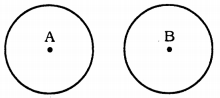There is no common point for these circles.

(2) Circles which touch externally :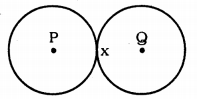For these pairs of circles, there is only one common point ‘x’.

(3) Circles which touch internally :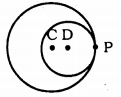These pair of circles touch internally. These have common end point ‘P’.

(4) Intersecting Circles :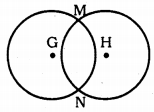For these pair of circles, M and N are two common points.
If we observe such circles we conclude that only two points are there.

Question 2.
Suppose you are given a circle. Give a construction to find its centre.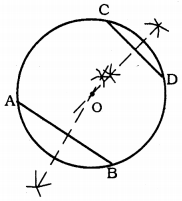Solution:
If a circle with any measurement, to find out centre of circle, steps are as follows :

1. Construct a circle with any radius.
2. Draw two chords AB and CD with any length.
3. Draw perpendicular bisector for AB and CD chords.
4. If these bisectors intersect each other when produced they meet at ’O’. O is the centre of the circle.

Question 3.
If two circles intersect at two points, prove that their centres lie on the perpendicular bisector of the common chord.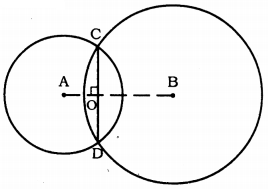Solution:
Consider two circles centred at point O and O’ intersecting each other at point A and B respectively.
Join AB. AB is the chord of the circle centred at O.
∴ Perpendicular bisector of AB will pass through O.
Again, AB is also the chord of the circle centred at O’.
∴ Perpendicular bisector ofAB will also pass through O’.
Clearly, the centres of these circles lie on the perpendicular bisector of the common chord.

All Chapter KSEEB Solutions For Class 9 Maths

—————————————————————————–

All Subject KSEEB Solutions For Class 9

*************************************************

I think you got complete solutions for this chapter. If You have any queries regarding this chapter, please comment on the below section our subject teacher will answer you. We tried our best to give complete solutions so you got good marks in your exam.

If these solutions have helped you, you can also share kseebsolutionsfor.com to your friends.

Best of Luck!!